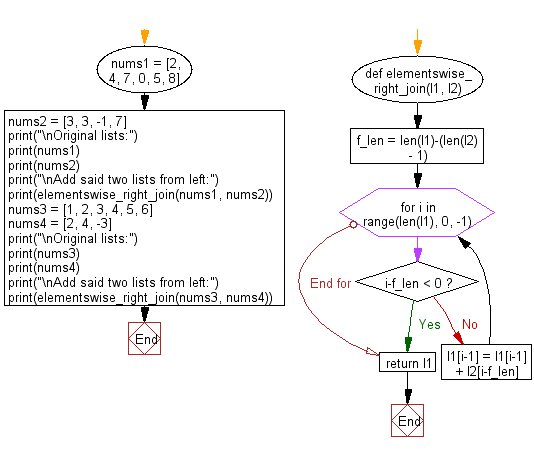﻿ Python: Add two given lists of different lengths, start from right - w3resource# Python: Add two given lists of different lengths, start from right

## Python List: Exercise - 156 with Solution

Write a Python program to add two given lists of different lengths, start from right.

Sample Solution:

Python Code:

``````def elementswise_right_join(l1, l2):
f_len = len(l1)-(len(l2) - 1)
for i in range(len(l1), 0, -1):
if i-f_len < 0:
break
else:
l1[i-1] = l1[i-1] + l2[i-f_len]
return l1

nums1 = [2, 4, 7, 0, 5, 8]
nums2 = [3, 3, -1, 7]
print("\nOriginal lists:")
print(nums1)
print(nums2)
print("\nAdd said two lists from left:")
print(elementswise_right_join(nums1, nums2))

nums3 = [1, 2, 3, 4, 5, 6]
nums4 = [2, 4, -3]
print("\nOriginal lists:")
print(nums3)
print(nums4)
print("\nAdd said two lists from left:")
print(elementswise_right_join(nums3, nums4))
```
```

Sample Output:

```Original lists:
[2, 4, 7, 0, 5, 8]
[3, 3, -1, 7]

Add said two lists from left:
[2, 4, 10, 3, 4, 15]

Original lists:
[1, 2, 3, 4, 5, 6]
[2, 4, -3]

Add said two lists from left:
[1, 2, 3, 6, 9, 3]
```

Flowchart:## Visualize Python code execution:

The following tool visualize what the computer is doing step-by-step as it executes the said program:

Python Code Editor:

Have another way to solve this solution? Contribute your code (and comments) through Disqus.

What is the difficulty level of this exercise?

Test your Python skills with w3resource's quiz

﻿

## Python: Tips of the Day

Floor Division:

When we speak of division we normally mean (/) float division operator, this will give a precise result in float format with decimals.

For a rounded integer result there is (//) floor division operator in Python. Floor division will only give integer results that are round numbers.

```print(1000 // 300)
print(1000 / 300)```

Output:

```3
3.3333333333333335```